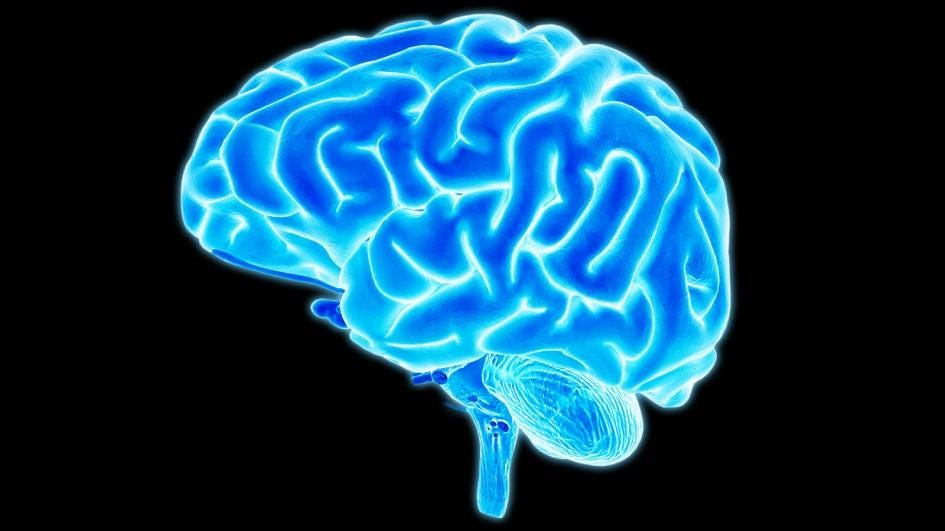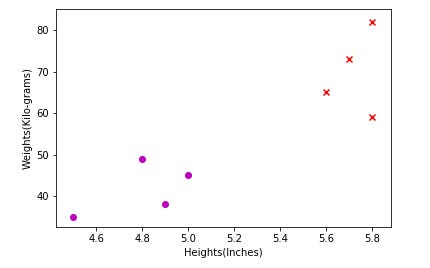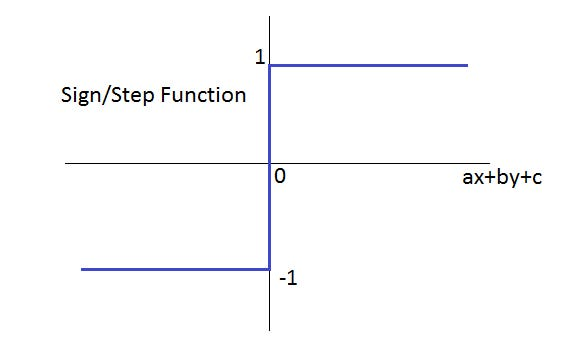# Simple Perceptron Training Algorithm:Explained

“I choose a lazy person to do a hard job. Because a lazy person will find an easy way to do it.”

-Bill Gates

We humans are so enthusiastic that we look at different things in nature and try to replicate it in our own way. Humans saw birds flying and wanted to invent something so that they could fly too. Many efforts were made, many inventions were invented, and eventually aeroplanes came into existence that enabled us to fly from one place to another. The source of all motivation was from mother nature. Similarly, there were efforts made to replicate the human brain. A number of researchers tried to understand the working of a human brain. After many years of research, Artificial Neural Networks were invented vaguely inspired from the biological neural networks inside our brain.

Human brain is really an amazing thing. It can identify objects, recognize patterns, classify things, and much more. What if a machine could do all this stuff? Wouldn’t that be cool? Today, as in 2018, we have come a long way in Artificial Intelligence. Many AI models are invented that could classify things, predict future, play games better than humans, and even communicate with us.

A neural network is a collection of neurons/nodes interconnected with each other through synaptic connections. An artificial neural network looks something like this.

The inputs to the neural network are fed to the input layer(the nodes in red color). Each node in a neural network has some function associated with it, each connection/edge has some weight value. The inputs are propagated from the input layer to the hidden layer (nodes in blue color). Finally, the outputs are received at the output layer(nodes in green color).

Today, lets build a perceptron model, which is nothing but a single node of a neural network.

Invented in 1957 by Frank Rosenblatt at the Cornell Aeronautical Laboratory , a perceptron is the simplest neural network possible: a computational model of a single neuron. A perceptron consists of one or more inputs, a processor, and a single output.

Lets understand the perceptron model with a simple classification problem.

Say, we have the input and output data,

Input::

x1 = Height of the person

x2 = Weight of the person

Output::

y = Gender(Male/Female)Red markers indicate Males and the markers in Magenta indicate Females

Our motive is to fit a decision boundary(a line) that separates all the male samples from the female samples. Well, we can do it by hand, try to find the equation of a line that separates both the classes. But, this is just a toy data, in real life applications, data is humongous, and we humans are too lazy to sit and go through each and every data point to find the equation of the decision boundary. Hence, we’ll use the perceptron model that’ll find the equation of the decision boundary for us. All we have to do is feed the input and output data for the model to train.

The general equation of a straight line is,

#### ax+by+c = 0 — — — eqn(1)

When we substitute the point P(x,y) in the equation, ax+by+c, it will give a value of 0(Since P lies on the line).

Similarly, when we substitute the point Q(x,y) in the equation, ax+by+c, it will give us a value greater than 0(Since Q lies above the line)., and

when we substitute the point R(x,y) in the equation ax+by+c, it will give us a value less than 0(Since R lies below the line).

Using this intuition, we can classify any point by substituting its value in the line equation,

If the resultant value is positive, the sample belongs to class Male(Y = 1),

if negative, the sample is a female sample(Y = -1).On Plotting the above property discussed, we get a function called the Sign function. This is the activation function that we are going to use. There are many more activation functions out there but for now, lets stick with sign function.

Let me rephrase eqn(1) as follows:

#### w0 + w1 * x1 + w2 * x2 = 0 — — — eqn(2)

where,

w0 = c ; w1 = a ; w2 = b ; x1 = x ; x2 = y.

We have the values of x1 and x2. We need the values of w0, w1, w2.

For mathematical convenience, lets vectorize eqn(2) as follows,

eqn(2)::

(or)

#### w0 * x0 + w1 * x1 + w2 * x2 = 0 … (where, x0 = 1)

Vector X::

Vector W::

we can define eqn(2) as dot product of vectors W and X

#### X.W = w0 * 1 + w1 * x1 + w2 * x2 = 0 — — — eqn(3)

If we successfully train our model and obtain optimum values of vector W, then eqn(3) should make classifications as follows…

if sample is a Male(Y = 1), then,

#### X.W > 0 — — — eqn(4)

if sample is a Female(Y = -1), then,

#### X.W < 0 — — — eqn(5)

Damn, now we got 2 constraints to satisfy(eqns 4 and 5),

Lets can combine eqns (4) and (5) as follows,

#### Y*(X.W) > 0 — — — eqn(6)

Where, Y = {1,-1}

If Y = 1;

1*(X.W) > 0

X.W > 0

If Y = -1;

1*(X.W) >0

X.W < 0

Alright, So we can conclude that our model correctly classifies the sample X if,

#### Y*(X.W) > 0 … (positive)

The sample is said to be misclassified if,

(or)

#### -Y*(X.W) > 0 — — — eqn(7) . . . (Misclassification Condition)

Now, to start off, we’ll randomly initialize the Weight vector W and for each misclassification we’ll update the weights as follows,

#### W = W + ΔW — — — eqn(8)

where ΔW is a small change that we will make in W.

Let’s Examine each misclassification case,

if Y = 1, and we got

X.W < 0, then

we need to update the Weights in such a way that,

X.(W+ ΔW) > X.W

Here, a good choice for ΔW would be η*X (positive value), i.e.,

ΔW = η*X — — — eqn(9)

if Y = 1, and we got

X.W < 0, then

we need to update the Weights in such a way that,

X.(W+ ΔW) > X.W

Here, a good choice for ΔW would be -η*X (negative value), i.e.,

ΔW = -η*X— — — eqn(10)

We can combine eqns(9 & 10) as,

ΔW = Y *(η*X) — — — eqn(11)

Therefore, eqn(8) implies,

W = W+Y *(η*X) — — — eqn(8)

Note: η is called the learning rate (usually greater than 0)

How did we get ΔW = Y*(η*X)? Keep reading to find out.

There’s an optimization algorithm, called the Gradient Descent. It is used to update the weights in case of misclassification. It does this by using a cost/loss function, that penalizes/tells us the loss in case of misclassification. Gradient Descent minimizes the cost function by gradually updating the weight values.

So, on to defining our cost function;

From eqn(7), we have the misclassification condition,

#### -Y*(X.W) > 0

Which means that “ -Y*(X.W) ” gives us a positive value for misclassification of input X.

So, let us assume our cost function(J) as,

#### Cost, J = -Y(X.W)

But, there’s one problem with this cost function, when the output is correctly classified,

Cost, J = -Y(X.W) = “Some negative value”…

but the cost function can’t be negative, so we’ll define our cost functions as follows,

If, -Y(X.W) > 0 ,

#### Cost, J = -Y(X.W) — — — eqn(12-a)

if, -Y(X.W) < 0 ,

#### Cost, J = 0 — — — eqn(12-b)

Note that we need to calculate the partial derivative of the cost function(J), with respect to weights W.

Partial Derivatives::

If, -Y(X.W) > 0 ,

#### ∂J/ ∂W = -Y*X

if, -Y(X.W) < 0 ,

#### ∂J/ ∂W = 0

Substituting the partial derivatives in gradient descent algorithm,

W = W – η*(∂J/ ∂W)

If, -Y(X.W) > 0 , (Misclassification)

#### W = W — η * (-Y*X) = W + η * (Y*X)

if, -Y(X.W) < 0 , (Correct Classification)

#### W = W — η * 0 = W …(No update in weights)

Hence, that’s how we got “W = W + η * (Y*X)” for cases of misclassification.

Finally, to summarize Perceptron training algorithm,

Perceptron models(with slight modifications), when connected with each other, form a neural network. Perceptron models can only learn on linearly separable data. If we want our model to train on non-linear data sets too, its better to go with neural networks.

Check out my github repository to see Perceptron training algorithm in action!!

Hope that made everything clear. Feel free to post your responses down in the response section below.

Follow me for more such Machine learning and Deep Learning articles.

Until then, don’t forget to feed your curiosity!!

Ciao 😉

Source: Deep Learning on Medium